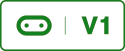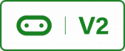# Bit Commander Game## Game Instructions

THIS CODE IS WRITTEN IN MICROPYTHON AND UPLOADED TO THE MICRO:BIT USING Mu.

Turn on Bit:Commander, press button A and B at the same time on the Micro:Bit to start a new game.

A number will be displayed on the Micro:bits screen, you must light up the same amount of NeoPixels using the control stick and press one of the 4 colour coded buttons. The button you press must be the same colour as the NeoPixels. For a correct answer you will get 1 point for an incorrect answer you will get -1 point. If your score is 0 it will not decrement any further therefore 0 is the lowest score you can get.

At the start of the game you will get 2500 ticks each tick is around 50mS giving you a game time of 50 seconds. When you get an answer correct you will be credited with more ticks. For each correct answer you get an extra 36 ticks which is around 1800 mS. Theoretically the game could last indefinitely if you answer the questions quick enough.

If you have a second Micro:Bit you can upload the display code that will display the score or the time or a mixture of both. To swap between time and score press the B button. To swap between the 2 every 5 seconds press the A button. The second Micro:Bit also outputs the score and time via the serial port so you can plug the second Micro:Bit into a computer and display the score and time simultaneously. I have included a processing sketch to show this.

## Game Code to be uploaded to the Micro:Bit plugged into the Bit:Commander

THIS CODE IS WRITTEN IN MICROPYTHON AND UPLOADED TO THE MICRO:BIT USING Mu.

Bit:Commander Game
``````#Micro:bit game designed for Bit:Commander

from microbit import *
import random
import music
import neopixel

np = neopixel.NeoPixel(pin13, 6)

yVal = 0

button_green = pin14
button_red = pin12
button_yellow = pin16
button_blue = pin15
dial = pin0

def setLEDs(start, end, red, green, blue):
for pixel_id in range(start, end):  # Start of for loop
np[pixel_id] = (red, green, blue)  # Code to be executed in the loop
np.show()

while True:
inProgress = False
isSelecting = False
isCorrect = False
ticks = 0
score = 0

while (inProgress == False):
display.show(Image.SMILE)
if(button_a.is_pressed() == True and button_b.is_pressed() == True):
inProgress = True
music.play(music.POWER_UP)
score = 0
ticks = 0
maxTicks = 2500 # about 50 seconds, each tick around 50 mS

#Game loop
while (inProgress == True):
target = random.randint(1,6)
random.seed(ticks)
display.show(str(target))
value = -1
direction = 0
targetSelected = False

colour = random.randint(0,3)
RGB = [0,0,0]
#set colour
if(colour == 0):
#red
RGB = [8,0,0]
elif(colour == 1):
#green
RGB = [0,8,0]
elif(colour == 2):
#blue
RGB = [0,0,8]
elif(colour == 3):
#yellow
RGB = [8,8,0]

isSelecting = True
#colour and number selection loop
while(isSelecting == True):
targetSelected = False
sleep(1)
ticks = ticks + 1
txPacket = str(score) + "," + str(ticks) + "," + str(maxTicks)
print(txPacket)

if (yVal > 512 and yVal > 25 + 512):
direction = 0 # 0 = up
yVal = (yVal - 512) * 2
elif (yVal < 25 + 512 and yVal > 512 - 25):
yVal = 0
direction = 0
elif (yVal < 512):
direction = 1
yVal = yVal * 2
if (yVal < 0):
yVal = 0
#LED light up stuff
setLEDs(0,6,0,0,0)
if(yVal < 20 and direction == 0): #Stick is centered turn lights off
setLEDs(0,6,0,0,0)
elif (yVal < 200):
if(direction == 0):
setLEDs(0,1,RGB,RGB,RGB)
value = 1
else:
setLEDs(0,6,RGB,RGB,RGB)
value = 6
elif (yVal < 400):
if(direction == 0):
setLEDs(0,2,RGB,RGB,RGB)
value = 2
else:
setLEDs(0,5,RGB,RGB,RGB)
value = 5
elif (yVal < 550):
if(direction == 0):
setLEDs(0,3,RGB,RGB,RGB)
value = 3
else:
setLEDs(0,4,RGB,RGB,RGB)
value = 4
elif (yVal < 725):
if(direction == 0):
setLEDs(0,4,RGB,RGB,RGB)
value = 4
else:
setLEDs(0,3,RGB,RGB,RGB)
value = 3
elif (yVal < 850):
if(direction == 0):
setLEDs(0,5,RGB,RGB,RGB)
value = 5
else:
setLEDs(0,2,RGB,RGB,RGB)
value = 2
elif (yVal < 1030):
if(direction == 0):
setLEDs(0,6,RGB,RGB,RGB)
value = 6
else:
setLEDs(0,1,RGB,RGB,RGB)
value = 1

if(value == target): # correct amount of lights lit up
#now check the buttons
if(colour == 0 and button_red.read_digital() == 1):
isCorrect = True
elif(colour == 1 and button_green.read_digital() == 1):
isCorrect = True
if(colour == 2 and button_blue.read_digital() == 1):
isCorrect = True
if(colour == 3 and button_yellow.read_digital() == 1):
isCorrect = True
if(isCorrect == True):
isSelecting = False

#incorrect choice
if(value != target):
isSelecting = False # new number and colour is chosen
music.play(music.JUMP_DOWN)
if(button_red.read_digital() == 1 and colour != 0):
isSelecting = False # new number and colour is c
music.play(music.JUMP_DOWN)
elif(button_green.read_digital() == 1 and colour != 1):
isSelecting = False # new number and colour is c
music.play(music.JUMP_DOWN)
elif(button_blue.read_digital() == 1 and colour != 2):
isSelecting = False # new number and colour is
music.play(music.JUMP_DOWN)
elif(button_yellow.read_digital() == 1 and colour != 3):
isSelecting = False # new number and colour is
music.play(music.JUMP_DOWN)

if (ticks > maxTicks):
inProgress = False
setLEDs(0,6,0,0,0)
music.play(music.PUNCHLINE)
display.scroll(str(score), 200)
isSelecting = False

if(isCorrect == False):
score = score - 1
if(score < 0):
score = 0

if(isCorrect == True):
score = score + 1
setLEDs(0,value,12,12,12)
music.play(music.BA_DING)
isCorrect = False
maxTicks = maxTicks + 36 #add 36 ticks for correct answer 1800 mS``````

Be aware that the above code uses 99% of the memory on the Micro:Bit so if you modify it you could have memory issues. Timings for the code are relative and not absolute this means that you have 2500 ticks at the start of the game however because when music is played and I use the sleep command it will take longer than 50 seconds for the game to complete. the sleep and music command are blocking commands that stop the Micro:Bit from carrying out other tasks while their executing. This can be demonstrated in the below video; notice how the time does not decrease because the Bit:Commander is busy playing the wrong answer tune.

This was an intentional design decision to demonstrate the affects of having your game loop bound to your logic and in the case of a computer game your render function. For a computer game it could mean that the faster your graphics card and frame rate the quicker the game clock runs. This was seen in Codemasters F1 2012, I believe that during online races people with better GPU’s were reporting better laptimes. In the world of robotics this could mean that your robot is not very responsive making it sluggish or causing it to crash.

## Display Code to be Uploaded to the Second Micro:Bit

THIS CODE IS WRITTEN IN MICROPYTHON AND UPLOADED TO THE MICRO:BIT USING Mu.

Remote Display Code
``````#Remote display for micro:bit game

from microbit import *

while True:
score = '0'
time = 1
maxTime = 1
display.show(score)
mode = 0 # 0 = score mode, 1 = time mode, 2 = both 5 second delay
subMode = 0

currentTicks = 0
lastTicks = 0
incomingList  = ["0","0","0"]

while (-1):
if(button_a.is_pressed()):
mode = 2
sleep(750)
if(button_b.is_pressed()):
if(mode == 0):
mode = 1
sleep(750)
else:
mode = 0
sleep(750)

sleep(30)
try:
elapsedPercent = (int(time)) / int(maxTime)
if incoming is not None:
incomingList = incoming.split(',')
print(incoming)
score = incomingList
time = incomingList
maxTime = incomingList
elapsedPercent = (int(time)) / int(maxTime)

if(mode == 2):
currentTicks = int(incomingList)
if (currentTicks - lastTicks > 360):
lastTicks = currentTicks
if(subMode == 0):
subMode = 1
else:
subMode = 0

if (mode == 0 or (mode == 2 and subMode == 0)):
if(int(score) < 10 and int(score) > -10):
display.show(score)
elif(int(score) < -10):
display.scroll(score, 100)
else:
display.scroll(score, 100)
if(mode == 1 or (mode == 2 and subMode == 1)):
if(elapsedPercent < 0.04):
display.show(Image('09999:99999:99999:99999:99999'))
elif(elapsedPercent < 0.08):
display.show(Image('00999:99999:99999:99999:99999'))
elif(elapsedPercent < 0.12):
display.show(Image('00099:99999:99999:99999:99999'))
elif(elapsedPercent < 0.16):
display.show(Image('00009:99999:99999:99999:99999'))
elif(elapsedPercent < 0.20):
display.show(Image('00000:99999:99999:99999:99999'))
elif(elapsedPercent < 0.24):
display.show(Image('00000:09999:99999:99999:99999'))
elif(elapsedPercent < 0.28):
display.show(Image('00000:00999:99999:99999:99999'))
elif(elapsedPercent < 0.32):
display.show(Image('00000:00099:99999:99999:99999'))
elif(elapsedPercent < 0.36):
display.show(Image('00000:00009:99999:99999:99999'))
elif(elapsedPercent < 0.40):
display.show(Image('00000:00000:99999:99999:99999'))
elif(elapsedPercent < 0.44):
display.show(Image('00000:00000:09999:99999:99999'))
elif(elapsedPercent < 0.48):
display.show(Image('00000:00000:00999:99999:99999'))
elif(elapsedPercent < 0.52):
display.show(Image('00000:00000:00099:99999:99999'))
elif(elapsedPercent < 0.56):
display.show(Image('00000:00000:00009:99999:99999'))
elif(elapsedPercent < 0.60):
display.show(Image('00000:00000:00000:99999:99999'))
elif(elapsedPercent < 0.64):
display.show(Image('00000:00000:00000:09999:99999'))
elif(elapsedPercent < 0.68):
display.show(Image('00000:00000:00000:00999:99999'))
elif(elapsedPercent < 0.72):
display.show(Image('00000:00000:00000:00099:99999'))
elif(elapsedPercent < 0.76):
display.show(Image('00000:00000:00000:00009:99999'))
elif(elapsedPercent < 0.80):
display.show(Image('00000:00000:00000:00000:99999'))
elif(elapsedPercent < 0.84):
display.show(Image('00000:00000:00000:00000:09999'))
elif(elapsedPercent < 0.88):
display.show(Image('00000:00000:00000:00000:00999'))
elif(elapsedPercent < 0.92):
display.show(Image('00000:00000:00000:00000:00099'))
elif(elapsedPercent < 0.96):
display.show(Image('00000:00000:00000:00000:00009'))
elif(elapsedPercent < 1):
display.show(Image('00000:00000:00000:00000:00000'))
else:
display.show(Image('99999:99999:99999:99999:99999'))
except:
pass``````

### Processing Application

THIS CODE IS WRITTEN IN JAVA AND EXECUTED USING PROCESSING 3

Processing is a great application where you can receive data from your project and display it in a meaningful way on you computer screen or phone. It can be downloaded from the following link;

Applications are written in Java and it’s a great tool to display telemetry data from robots and to send commands. I have included a simple program below that will enable you to connect the display or game Micro:Bit to your computer and have both the time and score displayed in real time. You could expand the program to include stats such as reaction time and save high scores to a database or text file.

The code for this processing application is shown below;

``````import processing.serial.*;

Serial mySerial;

int score = 0;
int ticks = 0;
int maxTicks = 0;
String incomingData = null;
int CR = 10; //Carage return

void setup()
{
size(400,200);
String myPort = Serial.list() ; //change this for your serial port
//this is comm port 5 for me not sure why!!!!
//remember the display will only transmit serial data when a game is in progress!!!!
mySerial = new Serial(this, myPort, 115200);
PFont f = createFont("Georgia", 64);
textFont(f);
textSize(32);
text("Game Not Started", 0, 50);
}

void draw()
{

while(mySerial.available() > 0)
{

if (incomingData != null) //Serial port has data on it lets process it
{
background(0); //update background
String[] data = split(incomingData, ',');
Double timeLeft = 0.0d;
Double a = 0.0d;
Double b = 0.0d;

try
{
data = data.replaceAll("\"","3");
a = Double.parseDouble(data);
b = Double.parseDouble(data);
timeLeft = (a - b);

}
catch (Exception e)
{
println(e);
}

timeLeft = (timeLeft * 20) / 1000;

text("Score", 0, 50);
text("Time Left", 200, 50);
text(data, 25, 100);
text(Double.toString(timeLeft), 220, 100);
}
}

}``````

PLEASE NOTE: You will have to amend line 14 with the COMM port that you’re using on your computer.

All the code from this example can be downloaded from BC_Game.zip or the resources section.